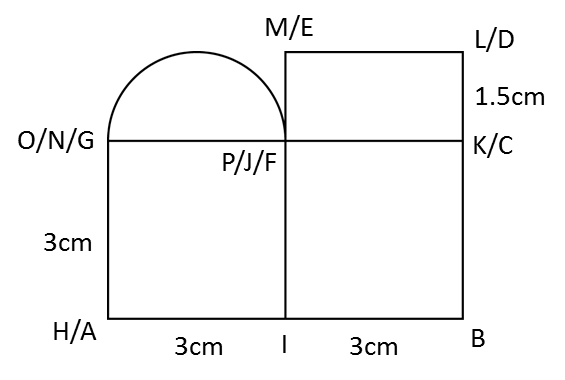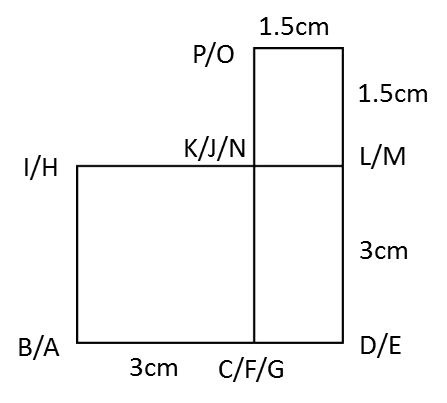# 10.3 SPM Practice (Long Questions)

Question 3:The diagram above shows a right prism attach to a cuboid at one of its plane. ABCG and CDEF, IJNH and KLMJ are horizontal planes, ABIH, AGNH and GCJN are vertical planes while IBCJ is an inclined plane. HA = 3 cm, BC = 3 cm, CD = 1.5 cm, HI = NJ = JK = 3 cm. Draw to full scale

(a) The elevation of the solid on a vertical plane parallel to AB as viewed from X.(b) A solid semi-cylinder is joined to the solid in A as show in the diagram above. JPON is a vertical plane and JP = 1.5 cm. Draw to full scale
i. The plan of the solid
ii.   The elevation of the solid on a vertical plane parallel to BCD as viewed from Y.

Solution:

(a)(b)(i)(b)(ii)